## Analog IC Design Course Homework 2 SIMetrix Edition

morreale Tuesday 02 of November, 2021

## Models

Public domain model files were provided for the homework assignments from the course website. Simulation examples with model files were also provided for LTspice and SIMetrix SPICE simulators. These educational models have limited documentation. The table below shows a summary of properties of the models.

Model Origin Type Level Category Variations Vt
0.8 um CMOS PTM .model 3 Planar unknown unknown
0.35 um CMOS Probably PTM .model 49 Planar SS,TT,FF unknown
0.18 um CMOS Probably PTM .model 49 Planar SS,TT,FF unknown
45 nm CMOS PTM .model 54 Planar SS,TT,FF vtl
LTspice 45 nm CMOS PTM .model 54 Planar SS,TT,FF vtl
SIMetrix example 45 nm CMOS PTM .subckt 14 Planar TT vtl
SIMetrix 7nm FinFET Modified PTM .subckt 72 FinFET TT lvt, rvt, slvt, sram

Various flavors of the NMOS and PMOS transistors are provided in the models based on threshold voltage Vt, and on process variations. These include:

• low Vt cells (lvt)
• regular Vt cells (rvt)
• super low Vt cells (slvt)
• low threshold High-speed (vtl)
• Low leakage (sram)
• SS: Slow NMOS, Slow PMOS
• TT: Typical NMOS, Typical PMOS
• FF: Fast NMOS, Fast PMOS

The models all appear to be generated from or are a modification of models produced by Predictive Technology Model (PTM), and are used to make standard cell logic gates.

For homework 2, I repeat homework 1 with the 7 nm FinFET model using the SIMetrix simulator. The default values of nmos_lvt 7 nm transistor model are set to NFIN=5 (5 fins), NFGR=1 (1 finger), and L=50n (50 nm length). The frequency response is terrible with gain of 7.7 at 1 MHz, and a unity-gain bandwidth of 13.1 MHz. The number of fingers can be increased to 1, 2, 5, 10, 20, 50, 100, etc. using the `Edit Device Properties` panel. The number of fins and parallel devices steps in this order too. Length steps this way as well and includes the nano multiplier (i.e. 1n, 2n, 10n ,20n, 50n, ...).

The nmos_lvt performance improves significantly with L=20n, NFIN=5, NFGR=1 with a gain of 23.1 and a unity-gain bandwidth of 5.6 GHz. Better yet, with L=20n, NFIN=5, NFGR=5 the gain is 21.8 and a unity-gain bandwidth of 360.3 GHz. The table below shows the performance for various model parameter settings.

Model L NFIN NFGR Gain Unity Gain BW
nmos_lvt 50 nm 5 1 7.7 13.1 MHz
nmos_lvt 20 nm 5 1 23.1 5.6 GHz
nmos_lvt 20 nm 5 5 21.8 360.3 GHz
nmos_lvt 20 nm 3 1 4.9 41.0 GHz
nmos_lvt 10 nm 3 1 5.9 41.0 GHz

One of the professors associated with the course indicated that the 7 nm transistor model is only valid for a gate length of 20 nm. There was no other guidance on the models so I've elected to use the following model parameters for these models to use three fins, one finger and a 20 nm gate length for this homework, and are shown in the table below. This selection is based on reference  and with background from the following references      .

Model L NFIN NFGR Gain Unity Gain BW
nmos_lvt 20 nm 3 1 4.9 41.0 GHz

Thus for question 3.2a, 3.2b, 4.2a, and 4.2b, gate length L=90 and L=45 are likely too long for the 7 nm nmos_lvt model and have been replaced with L=20 and L=10, respectively to stay in the estimated operating range of the model.

## Editing Component Properties

To make editing component properties easier use `File -> Options -> General` and check the `Enable GUI property edits` check box as shown below.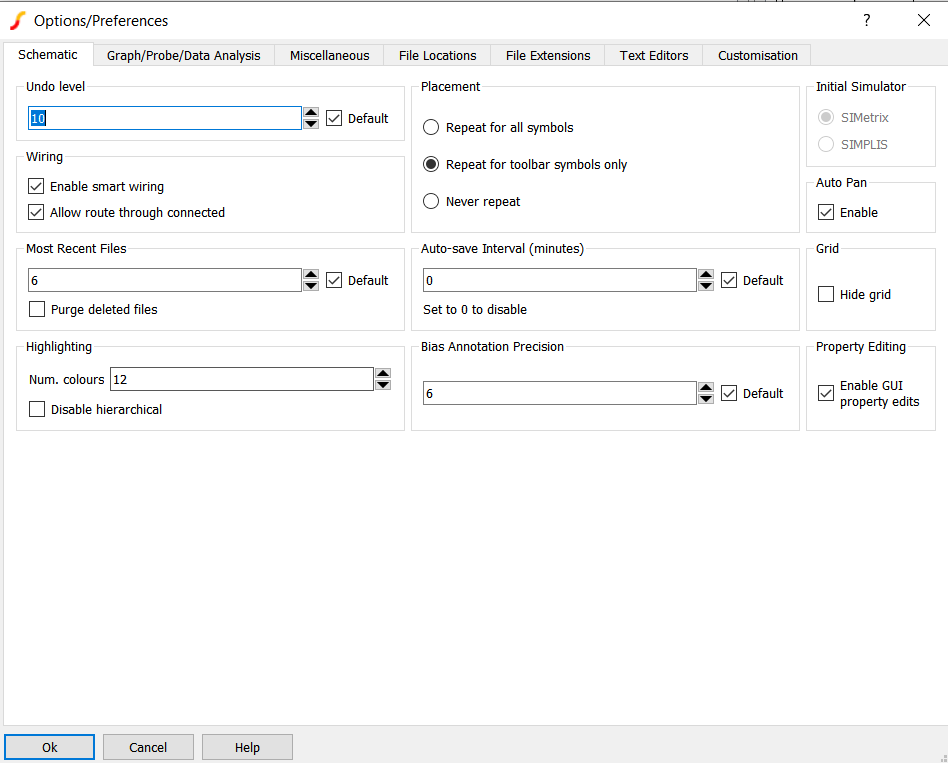## Problem 3: Repeat homework 1 & 2 with FinFET (or SOI models if you have them available). What differences and similarities are observed?

### 3.1a. plot I‐V characteristics

The schematic with a nmos_45 nm transistor used in Problem 1 and 2, and a 7 nm nmos_lvt transistor along with the IV curves for both transistors are shown below. The top plot shows Id vs VDS for the nmos_45 nm transistor with L=45nm, W=1u, and NFGR=5, and the bottom plot shows the 7 nm FinFET nmos_lvt transistor with L=20nm, NFGR=1, and NFIN=3.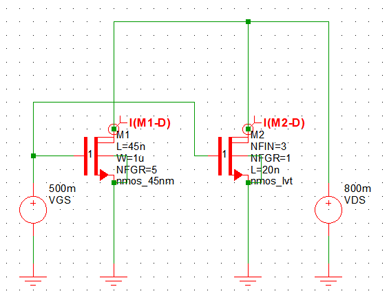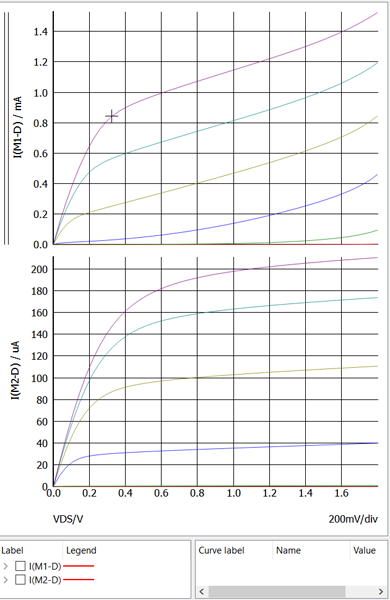### 3.1b. Extract Vt with VDS = 10mV (extrapolating linear part of the ID vs VGS plot)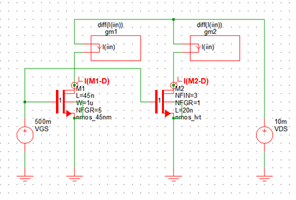Vt at VDS=10 mV is estimated to be 390 mV for the nmos-45nm transistor and 230 mV for the 7 nm FinFET.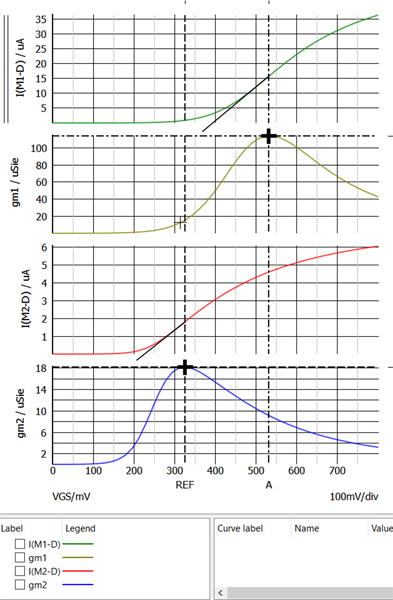### 3.1c. Extract Vt with VDS = 0.8V (extrapolating the linear part of the gm vs. VGS plot).

Vt at VDS=800 mV is estimated to be 270 mV for the nmos-45nm transistor and 230 mV for the 7 nm FinFET.### 3.1d. Compare results from parts (b) and (c ). What's the difference? What might explain this?

The decrease in Vt with increasing VDS is an indication of Drain Induced Barrier Lowering (DIBL) and can be seen in the nmos-45nm transistor and is estimated to be (390 - 270 mV/(10 - 800 mV)= -152 mV/V. My measurements for the 7 nm FinFET are not accurate enough to calculate the a value for DIBL but it is very small as the device shows very little variation in Vt with increasing VDS.

### 3.1e. Plot I‐V characteristics on a semilog scale. What is the subthreshold slope? Is GIDL evident?

The subthreshold slope for the 45 nm and 7 nm nmos transistors are shown below using Id vs VGS. The Subthreshold voltage for the 45 nm NMOS transistor is 100.7 mV/decade and the subthreshold voltage for the 7 nm NMOS transistor is 62.8 mV/decade. Gate Induced Drain Leakage (GIDL) isn't present in either transistor because Id doesn't increase at low VGS voltages.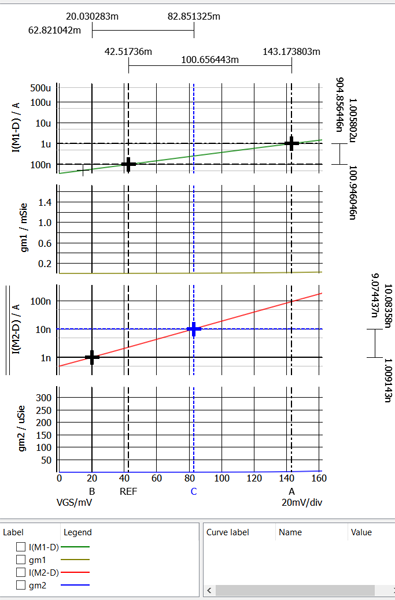### 3.1f. Plot gm/ID vs. VGS (with VDS = 0.8V). How does the value of obtained from plot (i.e. in subthreshold) compare with the subthreshold slope in part (e)?

The subthreshold slope for the 45 nm and 7 nm nmos (bottom) transistors are shown below using gm/ID vs. VGS. The Subthreshold voltage for the 45 nm NMOS transistor is 98.8 mV/decade (top trace) and the subthreshold voltage for the 7 nm NMOS transistor is 62.4 mV/decade (bottom trace). This method shows a slightly smaller subthreshold slope measurement.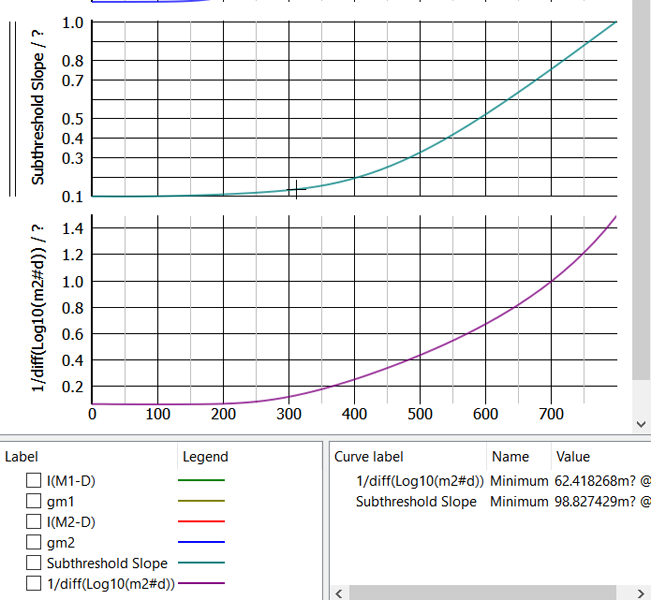### 3.2a. Find intrinsic gain of a transistor with L=45nm. What operating point maximizes the gain? How does this change with L=90nm?

The gate lengths for this problem have been revised to 10 nm and 20 nm because the 7nm nmos_lvt model does not seem to work well with gate lengths greater than 20 nm.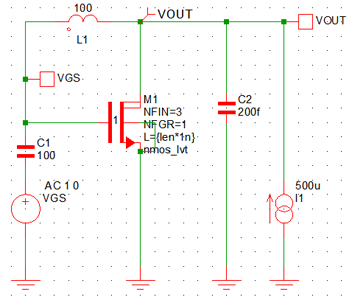The intrinsic gain was extracted from the AC analysis plot below and was found to be 4.9 for the L=20 nm NMOS transistors and the 5.9 for the L=10 nm NMOS transistor at 1 MHz. The model operation is suspect because the 7 nm nmos_lvt model with L=10n provides more gain than the model with L=20n. The longer gate length L=20 should produce more gain [^8] (see pg 38).

### 3.2b. What is the maximum unity‐gain bandwidth achievable when driving a load capacitance of 200 fF with a common‐source transistor while consuming 0.5mW from a 1‐V supply?

The unity gain bandwidth of the L=20 nm NMOS transistor is 186.7 MHz and the L=10 nm NMOS transistor is 183.7 MHz with NFGR=1 and NFIN=3.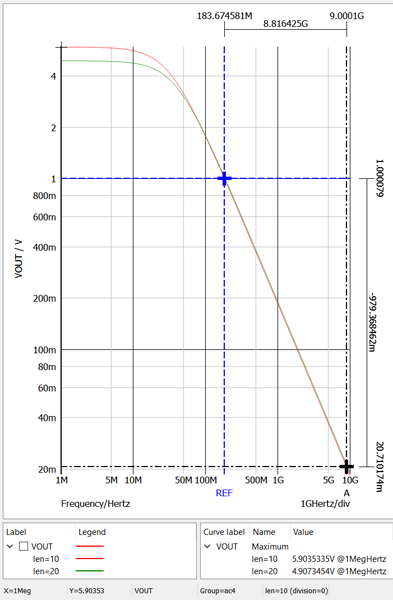### Summary

Summary of the transistor performance is shown in the table below.

Parameter nmos_45nm L=90 nm nmos_45 L=45 nm 7 nm nmos_lvt L=20 nm 7 nm nmos_lvt L=10 nm Units
Vt at VDS=10 mV, Id 390 230 mV
Vt at VDS=800 mV, gm 270 230 mV
Subthreshold at VDS=800 mV, Id 100.7 62.8 mV
Subthreshold at VDS=800 mV, gm 98.8 62.4 mV
n, at VDS=800 mV, Id 1.66 1.05
n at VDS=800 mV, gm 1.68 1.05
Gain at 10 MHz 27.4 5.0 4.9 5.9
Unity Gain Bandwidth 941.7 1307.8 183.7 183.7 MHz

## Problem 4: Repeat homework 1 with two stacked transistors using the same technology as 3 above. Compare the results.

### 4.1a. plot I‐V characteristics

The schematic with the two series 7 nm NMOS transistors and IV curve for Id vs VDS are shown below. The body connection of M1 was connected to its source out of habit, but should have been connected to ground as shown in the schematic below. The results only changed slightly. n -> 1 for the 7 nm nmos-lvt transistors. Lower n reduces or eliminates body effects, improves subthreshold slope, and improves gm/ID (see lecture 2 slide 27).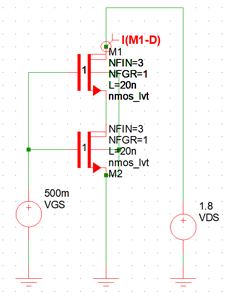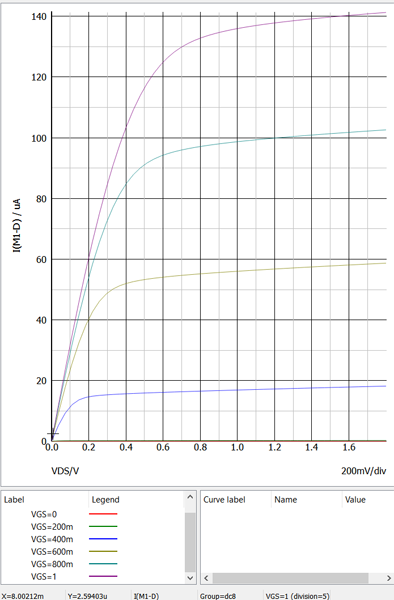### 4.1b. Extract Vt with VDS = 10 mV (extrapolating linear part of the ID vs VGS plot)

Vt at VDS=10 mV is estimated to be 230 mV for the series 7 nm FinFET.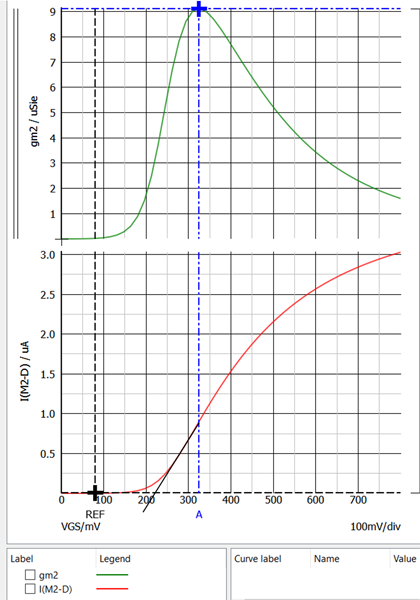### 4.1c. Extract Vt with VDS = 0.8V (extrapolating the linear part of the gm vs. VGS plot).

Vt at VDS=800 mV is estimated to be 230 mV for the series 7 nm FinFET.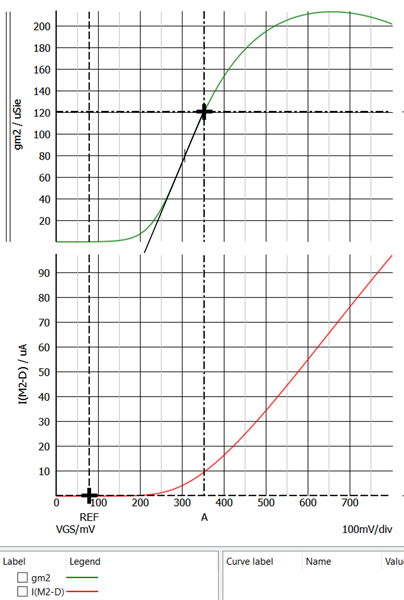### 4.1d. Compare results from parts (b) and (c ). What's the difference? What might explain this?

The 7 nm FinFET shows Very little variation in Vt with increasing VDS so no appreciable DIBL is present in this transistor.

### 4.1e. Plot I‐V characteristics on a semilog scale. What is the subthreshold slope? Is GIDL evident?

The subthreshold slope for the series 7 nm NMOS transistors is shown below using Id vs VGS. The Subthreshold voltage for the series 7 nm NMOS transistor is 62.0 mV/decade. Gate Induced Drain Leakage (GIDL) isn't present in these transistors because Id doesn't increase at low VGS voltages.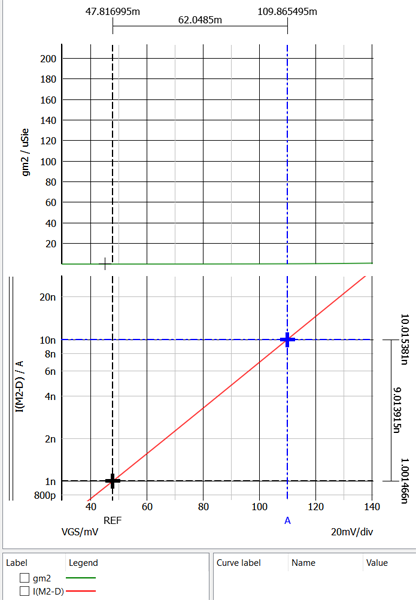### 4.1f. Plot gm/ID vs. VGS (with VDS = 0.8 V). How does the value of obtained from plot (i.e. in subthreshold) compare with the subthreshold slope in part (e)?

The subthreshold slope for series 7 nm nmos transistors is shown below using gm/ID vs. VGS. The Subthreshold voltage for the series 7 nm transistors is 61.7 mV/decade. This method shows a slightly smaller subthreshold slope measurement.### 4.2a. Find intrinsic gain of a transistor with L=45nm. What operating point maximizes the gain? How does this change with L=90nm?

Again, the gate lengths for this problems have been revised to 10 nm and 20 nm because the 7nm nmos_lvt model does not seem to work well with gate lengths greater than 20 nm.

The intrinsic gain was extracted from the AC analysis plot below and was found to be 1.00 for the L=20 nm NMOS transistors at 1.2 kHz, and the 83.4 for the L=10 nm NMOS transistor at 35 kHz.### 4.2b. What is the maximum unity‐gain bandwidth achievable when driving a load capacitance of 200 fF with a common‐source transistor while consuming 0.5mW from a 1‐V supply?

The unity gain bandwidth of the NMOS transistor with L=20 is 52.9 KHz and has some gain peaking at 32 kHz. The unity gain bandwidth of the NMOS transistor with L=10 nm is 109.6 MHz.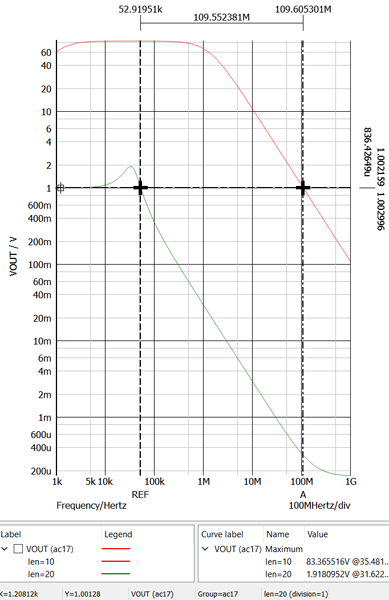### Summary

Summary of the serial transistor performance is shown in the table below.

Parameter 7 nm nmos_lvt L=20 nm 7 nm nmos_lvt L=10 nm Series 7 nm nmos_lvt L=20 nm Series 7 nm nmos_lvt L=10 nm Units
Vt at VDS=10 mV, Id 230 230 mV
Vt at VDS=800 mV, gm 230 230 mV
Subthreshold at VDS=800 mV, Id 62.8 62.0 mV
Subthreshold at VDS=800 mV, gm 52.4 61.7 mV
n, at VDS=800 mV, Id 1.05 1.04
n at VDS=800 mV, gm 1.05 1.04
Gain at 10 MHz/1 kHz 4.9 5.9 1.0 83.4
Unity Gain Bandwidth 183.7 183.7 .0529 109.6 MHz

## Problem 5: Plot ZDS vs. frequency for both transistor in 3 and the stacked-transistor in 4 Compare.

The schematics and plots for ZDS are shown below. The Maximum impedances for each simulation are shown in the table. For the series transistors, ZDS is twice ZDS of the single transistor response. The series transistor produce extra gain but reduce the bandwidth due to doubling the output impedance.

Parameter 7 nm nmos_lvt L=20 nm 7 nm nmos_lvt L=10 nm Series 7 nm nmos_lvt L=20 nm Series 7 nm nmos_lvt L=10 nm Units
ZDS at 1 MHz, VGS = 1 V 1533.4 1385.1 3066.8 2770.2 Ohms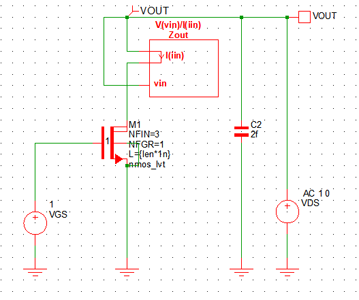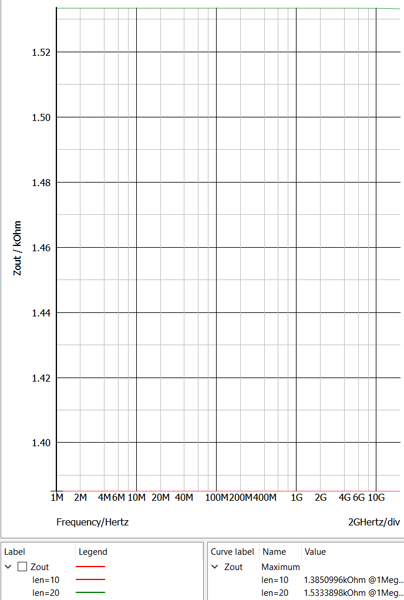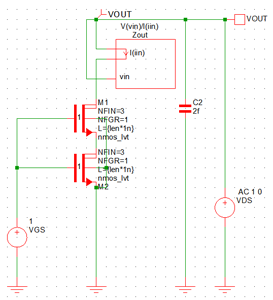## References

The references on the 7 nm transistor models were found on the ASAP7 ASU 7nm PDK website.

: L.T. Clark, V. Vashishtha, L. Shifren, A. Gujja, S. Sinha, B. Cline, C. Ramamurthya, and G. Yeric, ASAP7: A 7-nm FinFET Predictive Process Design Kit, Microelectronics Journal, vol. 53, pp. 105-115, July 2016.

: V. Vashishtha, L. Masand, A. Dosi and L. T. Clark, Systematic Analysis of the Timing and Power Impact of Pure Lines and Cuts Routing for Multiple Patterning, Proc. SPIE DTCO, 2017.

: V. Vashishtha, L. Masand, A. Dosi, and L. T. Clark, Design Technology Co-Optimization of Back End of Line Design Rules for a 7 nm Predictive Process Design Kit, Proc. ISQED, 2017.

: V. Vashishtha, M. Vangala, P. Sharma, and L. T. Clark, Robust 7-nm SRAM Design on a Predictive PDK, Proc. ISCAS, 2017.

: L. T. Clark, V. Vashishtha, D. M. Harris, Samuel Dietrich, and Zunyan Wang, Design Flows and Collateral for the ASAP7 7nm FinFET Predictive Process Design Kit, Proc. MSE, 2017.

: L. T. Clark and V. Vashishtha, , Design with sub-10 nm FinFET Technologies, Presented at CICC, 2017.

: V. Vashishtha, M. Vangala, and L. T. Clark, ASAP7 Predictive Design Kit Development And Cell Design Technology Co-Optimization, Proc. ICCAD, 2017.

: T. C. Carusone, D. A. Johns, and K. W. Martin, Analog Integrated Circuit Design, 2nd Edition. Wiley, 2011.